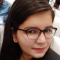## Recursive sum of digits of a number is prime or no in C++Sunidhi Bansal

Updated on 03-Nov-2021 07:40:46

Given an integer variable number as input. The goal is to calculate the sum of digits of the input number and check if that sum is prime or not. Do this till the obtained number with sum of digits becomes a single digit number. Check if that number is prime ... Read More

## Recursive sum of digits of a number formed by repeated appends in C++Sunidhi Bansal

Updated on 03-Nov-2021 07:36:06

Given two integers ‘number’ and ‘repeat’ as input. The goal is to calculate the sum of digits of the input number repeated ‘repeat’ number of times until the sum becomes a single digit. Do this till the obtained number with sum of digits becomes a single digit number. If the ... Read More

## Rotate a matrix by 90 degree in clockwise direction without using any extra space in C++Sunidhi Bansal

Updated on 03-Nov-2021 07:23:33

We are given a 2-D array that will be used to form a matrix pattern. The task is to rotate a matrix by 90 degrees in a clockwise direction such that the last row becomes the first column, second row becomes second column and first becomes third column and the ... Read More

## Refactorable number in C++Sunidhi Bansal

Updated on 03-Nov-2021 07:19:18

We are given an integer type value, let's say, number. The task is to check whether the given number is Refactorable or not. If yes print that the number is a refactorable number else print not possible.What is a Refactorable Number?A number is refactorable when it is divisible by its ... Read More

## Rearrange characters in a string such that no two adjacent are same in C++Sunidhi Bansal

Updated on 03-Nov-2021 07:13:17

We are given a string, let's say, str of any given length. The task is to rearrange the given string in such a manner that there won't be the same adjacent characters arranged together in the resultant string.Let us see various input output scenarios for this −Input − string str = ... Read More

## Rectangle with minimum possible difference between the length and the width in C++Sunidhi Bansal

Updated on 03-Nov-2021 07:09:52

Given an area of rectangle as input. The goal is to find the sides of a rectangle such that the difference between the length and breadth is minimum.Area of Rectangle = length * breadth.ExamplesInput − Area = 100Output −Side of Rectangle with minimum difference:Length = 10, Breadth = 10Explanation − Sides with area ... Read More

## Recursive program to insert a star between pair of identical characters in C++Sunidhi Bansal

Updated on 03-Nov-2021 07:06:09

Given a string str1 as input. The goal is to insert a ‘*’ between a pair of identical characters in the input string and return the resultant string using a recursive approach.If the input string is str1= "wellness" then output will be "wel*lnes*s"ExamplesInput − str1="happiness"Output − String after adding * : hap*pines*sExplanation − ... Read More

## Recursive Approach to find nth node from the end in the linked list in C++Sunidhi Bansal

Updated on 03-Nov-2021 06:59:53

Given a Singly linked list and positive integer N as input. The goal is to find the Nth node from the end in the given list using recursion. If the input list has nodes a → b → c → d → e → f and N is 4 then ... Read More

## Recursive approach for alternating split of Linked List in C++Sunidhi Bansal

Updated on 03-Nov-2021 06:56:48

Given a Singly linked list as input. The goal is to split the list into two singly linked lists that have alternate nodes of the original list. If the input list has nodes a → b → c → d → e → f then after the split, two sub-lists ... Read More

## Recursive insertion and traversal linked list in C++Sunidhi Bansal

Updated on 03-Nov-2021 06:53:40